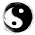## Wildcard Matching

Implement wildcard pattern matching with support for '?' and '*'.
'?' Matches any single character.
'*' Matches any sequence of characters (including the empty sequence).

The matching should cover the entire input string (not partial).

The function prototype should be:
bool isMatch(const char *s, const char *p)

Some examples:
isMatch("aa","a") â†’ false
isMatch("aa","aa") â†’ true
isMatch("aaa","aa") â†’ false
isMatch("aa", "*") â†’ true
isMatch("aa", "a*") â†’ true
isMatch("ab", "?*") â†’ true
isMatch("aab", "c*a*b") â†’ false

### Analysis:

For each element in s
If *s==*p or *p == ? which means this is a match, then goes to next element s++ p++.
If p=='*', this is also a match, but one or many chars may be available, so let us save this *'s position and the matched s position.
If not match, then we check if there is a * previously showed up,
if there is no *,  return false;
if there is an *,  we set current p to the next element of *, and set current s to the next saved s position.

e.g.

abed
?b*d**

a=?, go on, b=b, go on,
e=*, save * position star=3, save s position ss = 3, p++
e!=d,  check if there was a *, yes, ss++, s=ss; p=star+1
d=d, go on, meet the end.
check the rest element in p, if all are *, true, else false;

Note that in char array, the last is NOT NULL, to check the end, use  "*p"  or "*p=='\0'".

### Code(C++):

class Solution {
public:
bool isMatch(const char *s, const char *p) {
// Start typing your C/C++ solution below
// DO NOT write int main() function

const char* star=NULL;
const char* ss=s;
while (*s){
if ((*p=='?')||(*p==*s)){s++;p++;continue;}
if (*p=='*'){star=p++; ss=s;continue;}
if (star){ p = star+1; s=++ss;continue;}
return false;
}
while (*p=='*'){p++;}
return !*p;
}
};


### Code (Python):

class Solution:
# @param s, an input string
# @param p, a pattern string
# @return a boolean
def isMatch(self, s, p):
s_cur = 0;
p_cur= 0;
match = 0;
star = -1;
while s_cur<len(s):
if p_cur< len(p) and (s[s_cur]==p[p_cur] or p[p_cur]=='?'):
s_cur = s_cur + 1
p_cur = p_cur + 1
elif p_cur<len(p) and p[p_cur]=='*':
match = s_cur
star = p_cur
p_cur = p_cur+1
elif (star!=-1):
p_cur = star+1
match = match+1
s_cur = match
else:
return False
while p_cur<len(p) and p[p_cur]=='*':
p_cur = p_cur+1

if p_cur==len(p):
return True
else:
return False



1.2.nice thought!

3.This comment has been removed by the author.

4.very elegant.

5.请问这个和递归在时间复杂度上一样吗? 为什么递归过不了大集合?

1.时间复杂度不一样，每一个‘*’会产生一些分支，用没有优化过的递归是所有‘*’产生的分支都会被回溯直到发现match为止；而用这个方法只会回溯最后一个‘*’的分支，不会继续往上。把这个结构看成一棵树，这个方法只回溯最后一层。

2.那请问这个时间复杂度是怎么算的？

3.时间复杂度感觉是一样的吧

6.有一个问题不太懂，What if p is shorter then s? 第一个循环只看到了对＊s的保护，但是如果在s还没有结束的时候，p已经结束了，会发生error吗？

1.In my understanding, if current p is to its end, then *p ='\0' (remember the last element in a char array is '\0'). Therefore, the first two if condition will be false in the code, when the 3rd if (no star exists) is also false, the while loop will halt and return false.

2.不会, 因为p到最后了, 前两个判断会一直fail, 如果p中有*的话，会一直在第三个判断直到s结束, 如果没有*, 会经历第四个判断, 返回false.

7.这个算DP 吗？ 好像有点味道，但有不少常规的DP

8.9.s="aaab" p="*b" 这个case你的代码是false 是我理解错了么

1.I have run your test case "s=aaab p=*b", which gave the result "True".

Is there any misunderstanding?

2.This comment has been removed by the author.

10.这个不是dp吧，mn的复杂度啊。每次碰到不match的都要回退到上个*那里重来。

1.不是DP，这个解是个二叉树结构
if *p == '*'
isMatch(s, p) = isMatch(s, p + 1) || isMatch(s + 1, p + 1) || ... || isMatch(s + n, p+1)
= isMatch(s, p + 1) || isMatch(s + 1, p)
else
只有一个分叉 = isMatch(s+1, p+1)

这个算法的关键是当左子树再遇到＊的时候，上次遇到＊分裂出来的右子树就不用搜索了。
例如：s = aab... p = *a*b...
aab..., *a*b...
aab..., a*b... ab..., *a*b...
ab..., *b...

第二次遇到＊的时候 s = ab... p = *b...
如果s和p不匹配，那么上次遇到＊的右子树ab..., *a*b...也肯定不匹配（可以用反证法来证明）。
如果匹配，搜索左子树就能找到结果。

假设ab...和*a*b...匹配，那么ab...和*b...肯定匹配，和条件相反。

11.Hi Yu.

I have a question.In the below case,
char s[] = "acbacbac";
char p[] = "ac*a";

Should it be a matching ?

Thanks,
Di

1.There is no matching here.

12.Is it also possible that the string s has "*" and "?"?

1.The current c++ code might break if string s contains "*", but the algorithm is correct and the code can be fixed by reversing the first two if statements inside the while loop.

13.Great solution.
A clumsy but more details solution can be found here

14.very nice solution!
Sharp thought!

15.for p = "a*b", s = "a*ab", should we return true or false?

1.Should return true. At the beginning of while loop, should check *p == '*' first, to make sure the * in p is not skipped due to a * in s.

16.With
char s[] = "*a";
char p[] = "*aa";
your code returns false, which is not correct.

17.Isn't it false?

18.Yu, Just curious, how you code can match a sequence of char say 'aaaaa' with a asterisk '*' ?

1.Just because when there's a '*', and the pointer of p >= len(p), the pointer of s will move right, until reach the end, then we got True

19.This comment has been removed by the author.

20.Hey Yu, the 'char *ss' is really ingenious, which converted recursion into circulation. Thank you for your solution.

21.Why do you do ss++ if the star is not null?

22.23.24.I don't think it works for "*" and "abc". It works the other way around though.

25.This comment has been removed by the author.

26.I don't think the code works when string s has "*", it may be accepted on Leetcode but I donnot think it is correct, but it is really elegant.

1.Are you saying the input string itself having *?
that will be an overkill for the problem i think

27.This algorithm does not work with the following case:
aaa
*a

It should match, but it will generate mismatch

1.Good catch

28.29.http://www.theblisssolution.in/
Call us for fast support to this number.
91 72039 17285

30.Bliss Solution
Social media marketing (SMM) is continually progressing and adapting, becoming a for companies and brands. Social media platforms like Facebook, Twitter, LinkedIn, and Instagram can dynamically increase exposure and interest in your company. Search engines like Google and Bing are beginning to integrate updates, Tweets, profiles, and comments into their results pages, recognizing the importance of social interaction.